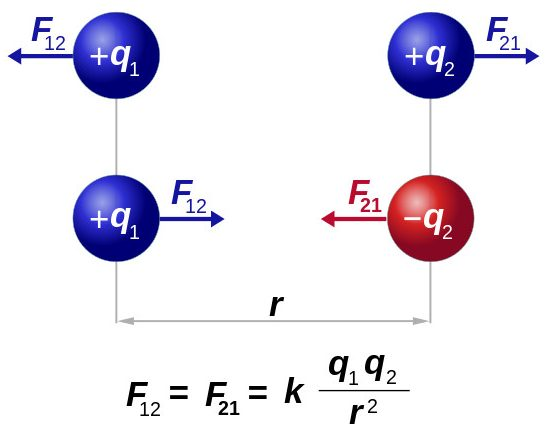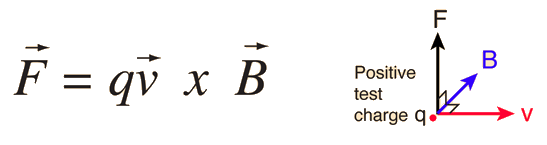# Equation For Strong Nuclear Force And Weak Nuclear Force

SNF Physics Equation Strength Newton QCD QM SR GR Function

5 replies to this topic

### #1Vmedvil

Vmedvil

Understanding

•• Members
•• 370 posts

Posted 27 September 2017 - 08:37 PM

Hello, I was wondering if there is equation for the Strong Nuclear Force Strength and Weak Nuclear Force Strength like Coulomb's Law or Newtonian Gravity. I would like it in Function of Force Form if possible. F(x) = (x) , It seems as if most of the other forces have equation such as this that explain it.

Newtonian Law of GravityCoulomb's Law of Electric ForceLorentz Law of MagnetismEdited by Vmedvil, 28 September 2017 - 01:42 AM.

### #2exchemist

exchemist

Creating

•• Members
•• 2847 posts

Posted 28 September 2017 - 01:25 AM

Hello, I was wondering if there is equation for the Strong Nuclear Force Strength and Weak Nuclear Force Strength like Coulomb's Law or Newtonian Gravity. I would like it in Function of Force Form if possible. F(x) = (x) , It seems as if most of the other forces have equation such as this that explain it.

Newtonian Law of GravityCoulomb's Law of Electric ForceLorentz Law of MagnetismThis is not my field, but there is an interesting discussion of this question here: https://physics.stac...g-nuclear-force

It does not seem as straight forward as electromagnetism or gravitation.

### #3Vmedvil

Vmedvil

Understanding

•• Members
•• 370 posts

Posted 28 September 2017 - 01:50 AM

This is not my field, but there is an interesting discussion of this question here: https://physics.stac...g-nuclear-force

It does not seem as straight forward as electromagnetism or gravitation.

That is very helpful that is in the form that I am seeking almost, Now what about the WNF. This though not as straight forward as EM or G is still much closer than any that I have found even though it is particle specific, meaning different for all particle pairs. The second equation in this page is much more general, based on the Yukama potential, add a 1/ Δx and it is Force, Now what about the WNF?

Edited by Vmedvil, 28 September 2017 - 04:52 AM.

### #4exchemist

exchemist

Creating

•• Members
•• 2847 posts

Posted 28 September 2017 - 02:11 AM

That is very helpful that is in the form that I am seeking almost, Now what about the WNF. This though not as straight forward as EM or G is still much closer than any that I have found even though it is particle specific, meaning different for all particle pairs. The second equation in this page is much more general, based on the Yukama potential, add a 1/ Delta Time and Mass and it is in force. Now what about the WNF?

I found the link by a google search. Why don't you now do that for the weak force and show us what you find?

### #5Vmedvil

Vmedvil

Understanding

•• Members
•• 370 posts

Posted 28 September 2017 - 02:16 AM

I found the link by a google search. Why don't you now do that for the weak force and show us what you find?

Lol, you googled and found that huh? Well, I googled and found this long equation for the WNF, I dunno I think you are better at this than I am.### #6exchemist

exchemist

Creating

•• Members
•• 2847 posts

Posted 28 September 2017 - 02:31 AM

Lol, you googled and found that huh? Well, I googled and found this long equation for the WNF, I dunno I think you are better at this than I am.Yes I've now had a look too, and see what you mean. I can't help you further I'm afraid.

### Also tagged with one or more of these keywords: SNF, Physics, Equation, Strength, Newton, QCD, QM, SR, GR, Function